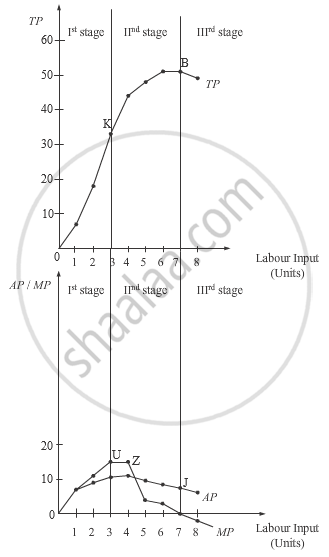# What Are the Different Phases in the Law of Variable Proportions in Terms of Total Product ? Give Reasons Behind Each Phase. Use Diagram. - Economics

What are the different phases in the Law of Variable Proportions in terms of Total Product ? Give reasons behind each phase. Use diagram.

#### Solution

The Law of Variable Proportions states that if more and more of variable factor (labour) is combined with the same quantity of fixed factor (capital), then initially the total product will increase but gradually after a point, the total product will become smaller and smaller.

​The following are the three phases (stages) of the Law of Variable Proportions.
Ist Stage: Increasing Returns to a Factor
This stages starts from the origin point O and continues till the point of inflexion (K) on the TP curve. During this phase, TP increases at an increasing rate and is also accompanied by rising MP curve (in figure ii). The MP curve attains its maximum point (U) corresponding to the point of inflexion. Throughout this stage, AP continues to rise.
Reasons for Increasing Returns to a Factor
Underutilisation of the fixed factor- In the first stage of production, there are not enough labour units to fully utilise the fixed factor. Therefore, the firm can increase its output just by combining more and more of labour inputs with the fixed factor, thereby; the output of the additional unit of labour (i.e. MP) tends to rise.
Division of labour- The increase in the labour input enables the division of labour, which further increases the efficiency and productivity of the labour.
Specialisation of labour- Due to the division of labour, specialisation of individual labour unit increases, which in turn raises the overall efficiency and productivity. Consequently, the MP curve rises and TP curve continues to rise.​

IInd Stage: Diminishing Returns to a Factor
This stage starts from point K and continues till point B on the TP curve. During this stage, the TP increases but at a decreasing rate and attains its  maximum point at B, where it remains constant. On the other hand (in the figure ii), the MP curve continues to fall and cuts AP from its maximum point Z, where MP equals AP. When TP attains its maximum point, corresponding to it, MP becomes zero. AP, in this stage initially rises, attains its maximum
point at Z and thereafter starts falling.
Reasons for Decreasing Returns to a Factor
Fuller utilisation of fixed factor- In this stage, the fixed factor is utilised to its maximum level as more and more of labour inputs are employed.
Imperfect substitutability between labour and capital- The variable factors are imperfect substitute for the fixed factor. Therefore, the firm cannot substitute labour for capital and as a result diminishing returns takes place.
Optimum Proportion/ Ideal Factor Ratio- The optimum proportion (or ideal factor ratio) is a fixed ratio in which the labour and capital inputs are employed. These factors will be the most efficient if they are employed as per the optimum proportion. If this proportion is disturbed (by combining more of labour inputs to the fixed units of capital), then the efficiency of the factors will fall, thereby leading to the diminishing returns to the factor.​

IIIrd Stage: Negative Returns to a Factor
This stage begins from the point B on the TP curve. Throughout this point, TP curve is falling and MP curve is negative. Simultaneously, the AP curve continues to fall and approaches the x-axis (but does not touch it). Like the first stage, this stage is also known as non- economic zone as any rational producer would not operate in this zone. This is because the addition to the total output by the additional labour unit (i.e. marginal product) is negative.This implies that employing more labour would not contribute anything to the total product but will add to cost of the production in form of additional wage. Hence, the cost of the additional labour input is greater than the benefit of employing it.
Reasons for Negative Returns to a Factor
Over utilisation of the fixed factors- In the third stage of production, the variable factor is in excessive relative to the fixed factors. This leads to the over utilisation of the fixed factor, thereby negative returns to a factor sets-in.
Negative Marginal Product- Throughout this stage the TP curve is continuously falling, consequently, the additional product by the additional unit of labour becomes negative. This implies that in this stage of production, the cost of employing labour is substantially higher than its contribution to the total product.
Problem of Management- With the increased number of labour units employed, it becomes hard for the management of the firm to efficiently manage them. Thus, due to the mismanagement and lack of responsibility, inefficiency is infused in the system.

Let us understand the various stages with the help of following schedule and graph.

 Units ofCapital Units ofLabour TP AP="Total Product"/"Units of Labour" MP=(ΔTP)/(ΔL 1 0 0 0 – 1 1 7 7 7 1 2 18 9 11 1 3 33 11 15 1 4 44 11 11 1 5 48 9.6 4 1 6 51 8.5 3 1 7 51 7.4 0 1 8 49 6.1 –2Concept: Law of Variable Proportions
Is there an error in this question or solution?